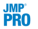Publication date: 11/10/2021

## Model Report

The Model Report shows a functional ANOVA table for the model parameter estimates. Specifically, it is an analysis of variance table where the variation is computed using a function-driven method.

Theta

Gaussian Process model parameter estimates. See Statistical Details for the Gaussian Process Platform.

Total Sensitivity

Sum of the main effect and all interaction terms for each factor. It is a measure of the amount of influence a factor and all its two-way interactions have on the response variable.

Total variation is the integrated variability over the entire experimental space.

Main Effect

The functional main effect of each factor is the integrated total variation due to that factor alone. The main effect is the ratio of the functional effect and the total variation for each factor in the model.

Interactions

Functional interaction effects are computed in a similar way to main effects.Categorical Input

When the model includes categorical factors, a correlation matrix for each categorical factor is provided. The off-diagonal entries correspond to Gaussian Process model parameter estimates. See Models with Categorical Predictors.

Mu and Sigma2

The mean and variance model parameters.

Nugget

The estimated nugget value. A nugget value is reported if you selected estimate nugget parameter in the Gaussian Process launch window. A nugget value is also reported if JMP has added a nugget parameter in order to avoid a singular covariance matrix.

-2LogLikelihood

The estimated value of twice the negative log-likelihood function. See Likelihood, AICc, and BIC in Fitting Linear Models.